﻿ Certain Properties of Generalized Fibonacci Sequence

### Certain Properties of Generalized Fibonacci Sequence

Yashwant K. Panwar, Mamta SinghOPEN ACCESSPEER-REVIEWED

## Certain Properties of Generalized Fibonacci Sequence

Yashwant K. Panwar1,, Mamta Singh2

1Department of Mathematics and MCA, Mandsaur Institute of Technology, Mandsaur (M. P.), India

2Department of Mathematical Sciences and Computer Application, Bundelkhand University, Jhansi (U. P.), India

### Abstract

In this study, we present certain properties of Generalized Fibonacci sequence. Generalized Fibonacci sequence is defined by recurrence relation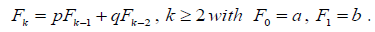. This was introduced by Gupta, Panwar and Sikhwal. We shall use the Induction method and Binet’s formula and give several interesting identities involving them.

• Panwar, Yashwant K., and Mamta Singh. "Certain Properties of Generalized Fibonacci Sequence." Turkish Journal of Analysis and Number Theory 2.1 (2014): 6-8.
• Panwar, Y. K. , & Singh, M. (2014). Certain Properties of Generalized Fibonacci Sequence. Turkish Journal of Analysis and Number Theory, 2(1), 6-8.
• Panwar, Yashwant K., and Mamta Singh. "Certain Properties of Generalized Fibonacci Sequence." Turkish Journal of Analysis and Number Theory 2, no. 1 (2014): 6-8.

 Import into BibTeX Import into EndNote Import into RefMan Import into RefWorks

### 1. Introduction

Fibonacci numbers are a popular topic for mathematical enrichment and popularization. The Fibonacci sequence is famous for possessing wonderful and amazing properties. The Fibonacci appear in numerous mathematical problems. Fibonacci composed a number text in which he did important work in number theory and the solution of algebraic equations. The book for which he is most famous in the “Liber abaci” published in 1202. In the third section of the book, he posed the equation of rabbit problem which is known as the first mathematical model for population growth. From the statement of rabbit problem, the famous Fibonacci numbers can be derived,This sequence in which each number is the sum of the two preceding numbers has proved extremely fruitful and appears in different areas in Mathematics and Science.

The Fibonacci sequence, Lucas sequence, Pell sequence, Pell-Lucas sequence, Jacobsthal sequence and Jacobsthal-Lucas sequence are most prominent examples of recursive sequences.

The Fibonacci sequence  is defined by the recurrence relation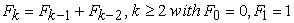(1.1)

The Lucas sequence  is defined by the recurrence relation(1.2)

The second order recurrence sequence has been generalized in two ways mainly, first by preserving the initial conditions and second by preserving the recurrence relation.

Kalman and Mena  generalize the Fibonacci sequence by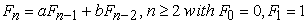(1.3)

Horadam  defined generalized Fibonacci sequence {Hn} by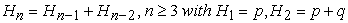(1.4)

where p and q are arbitrary integers.

Gupta, Panwar and Sikhwal , introduce generalized Fibonacci sequences. They focus only two cases of sequences {Vk}k0 and {Uk}k0 which generated by generalized Fibonacci sequences. They defined related identities of generalized Fibonacci sequences consisting even and odd terms. Also they present connection formulas for generalized Fibonacci sequences, Jacobsthal sequence and Jacobsthal-Lucas sequence. In , Gupta and Panwar have present identities involving common factors of generalized Fibonacci, Jacobsthal and Jacobsthal-Lucas numbers. In , Singh, Gupta and Panwar have present some addition identities of generalized Fibonacci sequences through Binet’s formula and Induction method. In , Panwar, Singh and Gupta have present generalized identities involving common factors of generalized Fibonacci, Jacobsthal and Jacobsthal-Lucas numbers. In , Panwar, Singh and Gupta derived many properties of generalized Fibonacci sequences through Binet’s formulas. Finally we present properties like Catalan’s identity, Cassini’s identity or Simpson’s identity and d’ocagnes’s identity for generalized Fibonacci sequences. In this paper, we present certain identities of Generalized Fibonacci sequence.

### 2. Generalized Fibonacci Sequence

Generalized Fibonacci sequence ([10, 12]), similar to the other second order classical sequences.

Generalized Fibonacci sequence is defined as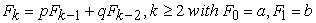(2.1)

where p, q, a & b are positive integers

For different values of p, q, a & b many sequences can be determined.

We will focus on one case of sequence {yk}k0 which generated in (2.1).

If p = a = 2, q = 1, b = 3, we get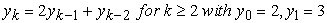(2.2)

The first few terms of {yk}k0 are 2, 3, 8, 19, 46, 111 and so on.

The explicit formula for yk is given as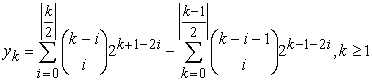(2.3)

### 3. Properties of Generalized Fibonacci Sequences

3.1. Binet’s Formula

In the 19th century, the French mathematician Binet devised two remarkable analytical formulas for the Fibonacci and Lucas numbers . In our case, Binet’s formula allows us to express the generalized Fibonacci numbers in function of the roots R1 & R2 of the following characteristic equation, associated to the recurrence relation (2.2)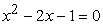(3.1)

Theorem 1: (Binet’s formula). The kth generalized Fibonacci number yk is given by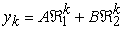(3.2)

where R1 & R2 are the roots of the characteristic equation (3.1) and R1 > R2 and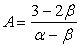and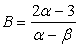.

Proof: we use the Principle of Mathematical Induction (PMI) on n. It is clear the result is true for k = 0 and k = 1 by hypothesis. Assume that it is true for r such that 0 ≤ r s + 1, then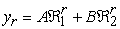(3.3)

It follows from definition of generalized Fibonacci numbers and equation (3.2)(3.4)

Thus, the formula is true for any positive integer.

3.2. Catalan's Identity

Catalan's identity for Fibonacci numbers was found in 1879 by Eugene Charles Catalan a Belgian mathematician who worked for the Belgian Academy of Science in the field of number theory.

Lemma 2: If r is a positive integer then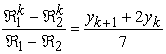(3.5)

Proof: Using the Principle of Mathematical Induction (PMI) on n, the proof is clear.

Theorem 3: (Catalan’s identity)(3.6)

Proof: By using Eq. (3.2) in the left hand side (LHS) of Eq. (3.6), and taking into account that R1R2 = 1 it is obtainedFinally, by using Eq. (3.5), the proof is clear.

3.3. Cassini's Identity

This is one of the oldest identities involving the Fibonacci numbers. It was discovered in 1680 by Jean-Dominique Cassini a French astronomer.

Theorem 4: (Cassini’s identity or Simpson’s identity)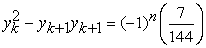(3.7)

Proof: Taking r = 1in Catalan’s identity the proof is completed.

3.4. d'Ocagne's Identity

Theorem 5: (d’ocagnes’s Identity)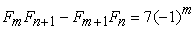(3.8)

where n is a natural number and m = n + 1.

Proof: Using the Principle of Mathematical Induction (PMI) on n, the proof is clear.

3.5. Limit of the Quotient of Two Consecutive Terms

A useful property in these sequences is that the limit of the quotient of two consecutive terms is equal to the positive root of the corresponding characteristic equation

Theorem 6: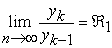(3.9)

Proof: Using Eq. (3.2)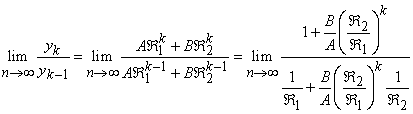and taking into account that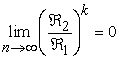since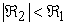, Eq. (3.9) is obtained.

3.6. Sum of the First Terms of the Generalized Fibonacci Sequence

Theorem 7: Let yk, be the kth generalized Fibonacci number then(3.10)

Proof: Using the Binet’s formula for the generalized Fibonacci numbers,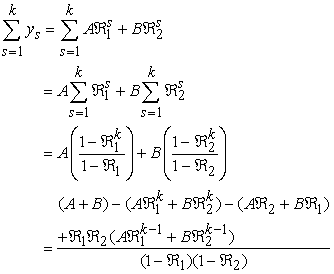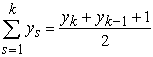This completes the proof.

3.7. Generating Function for the Generalized Fibonacci Sequence

Generating functions provide a powerful technique for solving linear homogeneous recurrence relations. Even though generating functions are typically used in conjunction with linear recurrence relations with constant coefficients, we will systematically make use of them for linear recurrence relations with non constant coefficients. In this paragraph, the generating function for generalized Fibonacci sequence is given. As a result, generalized Fibonacci sequence is seen as the coefficients of the corresponding generating function. Function defined in such a way is called the generating function of the generalized Fibonacci sequence. So,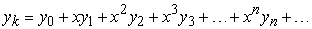and then,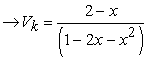(3.11)

### 4. Conclusion

In this study new generalized Fibonacci sequence have been introduced and studied. Many of the properties of these sequences are proved by simple algebra. In a compact and direct way many formulas of such numbers have been deduced.

### References

  A. F. Horadam, Basic Properties of Certain Generalized Sequence of numbers, The Fib. Quart, Vol. 3, No. 3, (1965), 161-176.In article  A. F. Horadam, The Generalized Fibonacci Sequences, The American Math. Monthly, Vol. 68, No. 5, (1961), 455-459.In article CrossRef  A. T. Benjamin and J. J. Quinn, Recounting Fibonacci and Lucas identities, College Math. J., Vol. 30, No. 5, (1999), 359-366.In article CrossRef  B. Singh, V. K. Gupta and Y. K. Panwar, Some Identities of Generalized Fibonacci Sequences, South pacific journal of Pure and Applied Mathematics, Vol. 1, no. 1, (2012), 80-86.In article  D. Kalman and R. Mena, The Fibonacci Numbers – Exposed, The Mathematical Magazine, Vol. 76, No. 3, (2003), 167-181.In article CrossRef  G. Udrea, A Note on the Sequence of A. F. Horadam, Portugalete Mathematica, Vol. 53, No. 2, (1996), 143-144.In article  N. N. Vorobyov, The Fibonacci numbers, D. C. Health and company, Boston, 1963.In article  S. Vajda, Fibonacci and Lucas numbers, and the golden section. Theory and applications, Chichester: Ellis Horwood limited (1989).In article  T. Koshy, Fibonacci and Lucas Numbers with Applications, A Wiley-Interscience Publication, New York, 2001.In article  V. K. Gupta, Y. K. Panwar and O. Sikhwal, Generalized Fibonacci Sequences, Theoretical Mathematics & Applications, Vol. 2, No. 2, (2012), 115-124.In article  V. K. Gupta and Y. K. Panwar, Common Factors of Generalized Fibonacci, Jacobsthal and Jacobsthal-Lucas numbers, International Journal of Applied Mathematical Research, Vol. 1, No. 4, (2012), 377-382.In article CrossRef  Y. K. Panwar, Generalized Fibonacci Sequences, LAP, Germany (2012).In article  Y. K. Panwar, B. Singh and V. K. Gupta, Generalized Fibonacci Sequences and Its Properties, Palestine Journal of Mathematics, Vol. 3, No. 1, (2014), 141-147.In article  Y. K. Panwar, B. Singh and V. K. Gupta, Generalized Identities Involving Common Factors of Generalized Fibonacci, Jacobsthal and Jacobsthal-Lucas numbers, International journal of Analysis and Application, Vol. 3, No. 1, (2013), 53-59.In article  Y. K. Panwar, V. K. Gupta and M. Singh, A Fibonacci-Like Sequence, LAP, Germany (2013).In article  Y. K. Panwar, B. Singh and V. K. Gupta, Generalized Fibonacci polynomials, Turkish Journal of Analysis and Number Theory. 2013, 1 (1), 43-47.In article